2023.0.11

• `public interface ILcdIntersectionMatrixPattern`
This interface defines a topological relationship between two shapes by imposing conditions on the intersection matrix of the two shapes.
Since:
10.0
`ILcdIntersectionMatrix`
• ### Field Summary

Fields
Modifier and Type Field and Description
`static ILcdIntersectionMatrixPattern` `CONTAINS`
Matrix pattern checking the topological relationship contains.
`static ILcdIntersectionMatrixPattern` `COVERED_BY`
Matrix pattern checking the topological relationship coveredBy.
`static ILcdIntersectionMatrixPattern` `COVERS`
Matrix pattern checking the topological relationship covers.
`static ILcdIntersectionMatrixPattern` `CROSSES`
Matrix pattern checking the topological relationship crosses.
`static ILcdIntersectionMatrixPattern` `DISJOINT`
Matrix pattern checking the topological relationship disjoint.
`static ILcdIntersectionMatrixPattern` `EQUALS`
Matrix pattern checking the topological relationship equals.
`static ILcdIntersectionMatrixPattern` `INTERSECTS`
Matrix pattern checking the topological relationship intersects.
`static ILcdIntersectionMatrixPattern` `OVERLAPS`
Matrix pattern checking the topological relationship overlaps.
`static ILcdIntersectionMatrixPattern` `TOUCHES`
Matrix pattern checking the topological relationship touches.
`static ILcdIntersectionMatrixPattern` `WITHIN`
Matrix pattern checking the topological relationship within.
• ### Method Summary

All Methods
Modifier and Type Method and Description
`boolean` `matches(ILcdIntersectionMatrix aIntersectionMatrix)`
Checks whether the topological relationship defined by this matrix pattern holds for the given intersection matrix.
• ### Field Detail

• #### DISJOINT

`static final ILcdIntersectionMatrixPattern DISJOINT`

Matrix pattern checking the topological relationship disjoint. Two shapes are disjoint if they have no points in common. This relation is the negation of the `INTERSECTS` relation.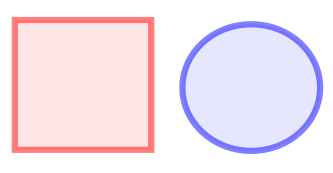In the example in the image, the red and blue shape are disjoint.

In terms of the DE-9IM matrix, this relation is true in case the intersection matrix matches one of the following patterns:
• `FF*FF****`
• #### INTERSECTS

`static final ILcdIntersectionMatrixPattern INTERSECTS`

Matrix pattern checking the topological relationship intersects. Two shapes intersect if they have at least one point in common. This relation is the negation of the `DISJOINT` relation.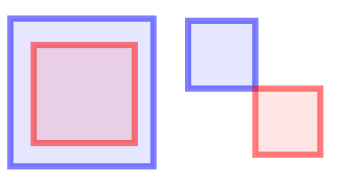In the two examples in the image, the red and blue shapes intersect.

In terms of the DE-9IM matrix, this relation is true in case the intersection matrix matches one of the following patterns:
• `T********`
• `*T*******`
• `***T*****`
• `****T****`
• #### CONTAINS

`static final ILcdIntersectionMatrixPattern CONTAINS`

Matrix pattern checking the topological relationship contains. This relation is the inverse of the `WITHIN` relation. The first shape contains the second shape if the following conditions are fulfilled:

• their interiors intersect
• the exterior of the first shape does not intersect the interior nor the boundary of the second shape

Note that the first condition implies that a polygon does not contain its own contour (a closed polyline). This illustrates the subtle difference between the contains relation and the `COVERS` relation , since a polygon covers its own contour.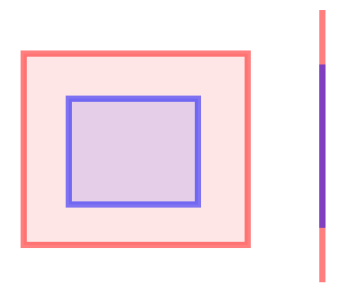In the two examples in the image, the red shape contains the blue shape.

In terms of the DE-9IM matrix, this relation is true in case the intersection matrix matches one of the following patterns:
• `T*****FF*`
• #### WITHIN

`static final ILcdIntersectionMatrixPattern WITHIN`

Matrix pattern checking the topological relationship within. This relation is the inverse of the `CONTAINS` relation. The first shape is within the second shape if the following conditions are fulfilled:

• their interiors intersect
• the exterior of the second shape does not intersect the interior nor the boundary of the first shape

Note that the first condition implies that the contour of a polygon is not within the polygon. This illustrates the subtle difference between the within relation and the `COVERED_BY` relation, since the contour of a polygon is covered by the polygon.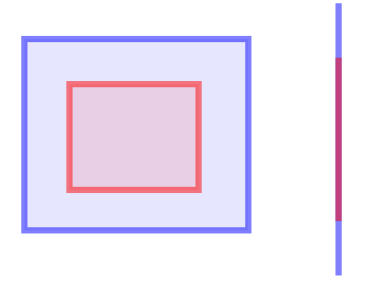In the two examples in the image, the red shape is within the blue shape.

In terms of the DE-9IM matrix, this relation is true in case the intersection matrix matches one of the following patterns:
• `T*F**F***`
• #### COVERS

`static final ILcdIntersectionMatrixPattern COVERS`

Matrix pattern checking the topological relationship covers. This relation is the inverse of the `COVERED_BY` relation. The first shape covers the second shape if the following conditions are fulfilled:

• both shapes intersect
• the exterior of the first shape does not intersect the interior nor the boundary of the second shape

Note the subtle difference with the `CONTAINS` relation.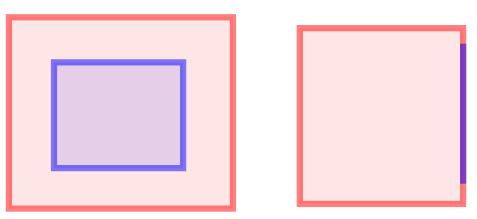In the two examples in the image, the red shape covers the blue shape.

In terms of the DE-9IM matrix, this relation is true in case the intersection matrix matches one of the following patterns:
• `T*****FF*`
• `*T****FF*`
• `***T**FF*`
• `****T*FF*`
• #### COVERED_BY

`static final ILcdIntersectionMatrixPattern COVERED_BY`

Matrix pattern checking the topological relationship coveredBy. This relation is the inverse of the `COVERS` relation. The first shape is covered by the second shape if the following conditions are fulfilled:

• both shapes intersect
• the exterior of the second shape does not intersect the interior nor the boundary of the first shape

Note the subtle difference with the `WITHIN` relation.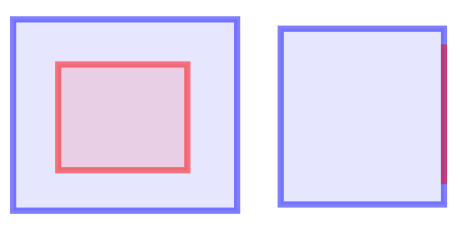In the two examples in the image, the red shape is covered by the blue shape.

In terms of the DE-9IM matrix, this relation is true in case the intersection matrix matches one of the following patterns:
• `T*F**F***`
• `*TF**F***`
• `**FT*F***`
• `**F*TF***`
• #### TOUCHES

`static final ILcdIntersectionMatrixPattern TOUCHES`

Matrix pattern checking the topological relationship touches. Two shapes touch each other if the following two conditions are fulfilled:

• their interiors have no point in common
• the boundary of one of the two shapes has at least one point in common with the other shape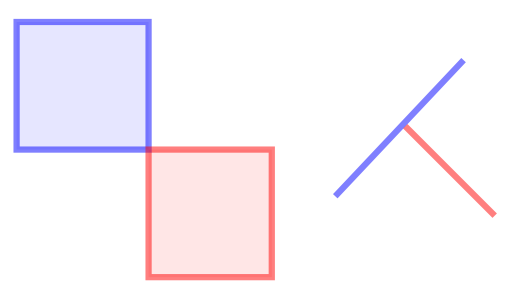In the two examples in the image, the red and blue shapes touch.

In terms of the DE-9IM matrix, this relation is true in case the intersection matrix matches one of the following patterns:
• `FT*******`
• `F**T*****`
• `F***T****`
• #### CROSSES

`static final ILcdIntersectionMatrixPattern CROSSES`

Matrix pattern checking the topological relationship crosses. Two shapes cross each other if the following three conditions are fulfilled:

• their interiors have at least one point in common
• the dimension of the intersection of their interiors is smaller than the dimension of the interior of the shape with the highest dimension
• neither shape lies completely inside the other shape

In practice, this means that:

• Two 1-dimensional shapes (such as polylines) cross if the intersection of their interiors has dimension 0.
• A 1-dimensional shape crosses a 2-dimensional shape if the interior of the 1-dimensional shape intersects both the interior and exterior of the 2-dimensional shape.
• A 0-dimensional shape (such as a point) never crosses another shape.
• Two 2-dimensional shapes never cross each other.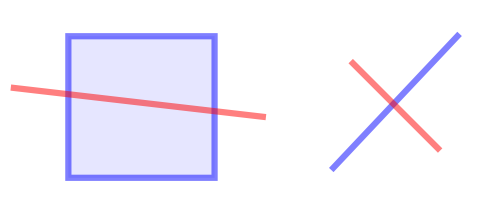In the two examples in the image, the red and blue shapes cross.

In terms of the DE-9IM matrix, this relation is true in case the intersection matrix matches one of the following patterns:
• `0********` for two 1-dimensional shapes
• `T*T******` for a 1-dimensional and a 2-dimensional shape
• `T*****T**` for a 2-dimensional and a 1-dimensional shape
• #### OVERLAPS

`static final ILcdIntersectionMatrixPattern OVERLAPS`

Matrix pattern checking the topological relationship overlaps. Two shapes overlap if the following conditions are fulfilled:

• their dimension is the same
• the intersection of their interiors has the same dimension as the shapes
• neither shape lies completely inside the other shape

This means that the overlap relation is always false for a:

• 0-dimensional shape (such as a point) and any other shape
• 1-dimensional shape and a 2-dimensional shape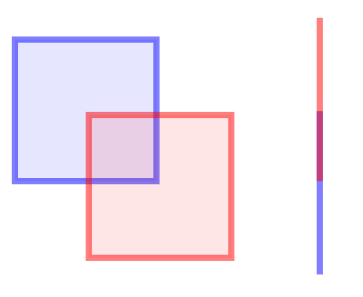In the two examples in the image, the red and blue shapes overlap.

In terms of the DE-9IM matrix, this relation is true in case the intersection matrix matches one of the following patterns:
• `1*T***T**` for two 1-dimensional shapes
• `T*T***T**` for two 2-dimensional shapes
• #### EQUALS

`static final ILcdIntersectionMatrixPattern EQUALS`

Matrix pattern checking the topological relationship equals. The equals relation is true for two shapes which have the same interior, boundary, and exterior.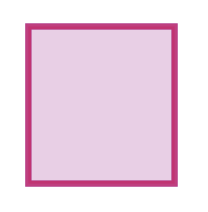In the example in the image, the red and blue shape are equal.

In terms of the DE-9IM matrix, this relation is true in case the intersection matrix matches one of the following patterns:
• `T*F**FFF*`
• ### Method Detail

• #### matches

`boolean matches(ILcdIntersectionMatrix aIntersectionMatrix)`
Checks whether the topological relationship defined by this matrix pattern holds for the given intersection matrix.
Parameters:
`aIntersectionMatrix` - the intersection matrix
Returns:
`true` if the binary topology relation defined by this matrix pattern holds for `aIntersectionMatrix`, false otherwise.